Next: 4. Abstract recurrent neural Up: 3. Mixture of local Previous: 3.4.2 Results

# 3.5 Discussion

Two local PCA mixture models were presented. The first model is an extension of Neural Gas to local PCA. The code-book vectors of Neural Gas are replaced by local PCA units. Two different variants were shown. One (NGPCA) uses the normalized Mahalanobis distance plus reconstruction error for the competition between units. The other one (NGPCA-constV) uses a modified error measure that ignores the volume of the ellipsoid associated with the local PCA.

The second model is an extension of the mixture of probabilistic PCA (MPPCA-ext). It contains three modifications: first, an initialization with Neural Gas; second, a neural network (RRLSA) to extract the local principal components, this network allows the addition of noise for each on-line presentation of a training pattern; third, a correction for units that have no patterns assigned to them.

Both models could successfully fit synthetic two- and three-dimensional training data, and they could be used to classify hand-written digits. No clear advantage of one model over the other could be observed. Both have advantages and disadvantages relative to each other.

NGPCA and NGPCA-constV worked on data with arbitrarily many dimensions; MPPCA-ext failed on the 784-dimensional data because of numerical instabilities. Furthermore, data distributions can be constructed (the two-lines distribution, figure 3.9) on which MPPCA-ext ends in a local optimum, but both NGPCA variants find the global optimum. Different from the Neural Gas initialization in MPPCA-ext, NGPCA considers the shape of the ellipses during annealing, and can therefore fit them to the distribution before the annealing cools down and gets trapped in a local optimum. However, the two-lines distribution is highly artificial, a sensorimotor distribution likely does not comprise two parallel or almost parallel planes that are also close to each other.

On the other hand, MPPCA-ext is less sensitive to the Neural Gas parameters (tmax,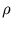(0),(tmax),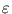(0), and(tmax)); for some distributions (especially the visuomotor model discussed in chapter 6), NGPCA depends on these parameters. For MPPCA-ext, standard parameters could be defined that worked for all tests in this thesis. Further, MPPCA-ext is mathematically more sound. It maximizes the likelihood of the data given an assumption on the density; NGPCA is heuristic. We could not prove that NGPCA optimizes any specific function. However, it seems to maximize the likelihood as well.

A disadvantage of NGPCA is that it may produce dead units that do not get updated anymore (figure 3.8.A). To avoid dead units, the variant NGPCA-const was introduced, which worked better on sparsely distributed data (figure 3.3). On the thin spiral distribution, however, NGPCA-constV resulted in a thin ellipsoid that protruded out of the spiral and connected distant parts (figure 3.8.C). This result occurred probably because here the normalization of the ellipsoid's volume had the opposite effect to the case of a huge ellipsoid; for ellipsoids with a tiny volume, the NGPCA error measure (3.2) results in a low weight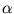, but the NGPCA-const error measure (3.10) is independent of the volume. Thus, for NGPCA-constV, chances are higher that patterns are assigned to the thin ellipsoid. For all NGPCA variants, these chances are highest in the direction of the ellipsoid's tips. Therefore, NGPCA-constV might attract distant patterns in these directions.

In this chapter, the advantages of just two of the three modification of MPPCA were visible. The good fit of the ring-line-square data and probably also the slight improvement of the digit classification result from the new Neural Gas initialization. For the tasks in this thesis, this initialization seemed to cure most problems with local optima associated with MPPCA. Therefore, MPPCA was preferred over those approaches that include annealing into the EM-iteration (Albrecht et al., 2000; Meinicke and Ritter, 2001) because they also include many training parameters. The second modification, the correction for empty units was helpful to fit sparse data distributions (figure 3.7). The advantage of the third modification, adding noise to increase the variance of each training pattern, was not shown, but it proved to be necessary for approximating sensorimotor distributions (chapter 6).

The new extension of Neural Gas to local PCA is clearly better on the digit classification then Neural Gas (with the same number of free parameters) and PCA alone. Despite the apparent complexity of the task, a single linear model describes each digit quite well (resulting in 4.85% miss-classified digits). Thus, digit-classification does not seem to be an ideal test for a local PCA mixture model, though it is a popular test (Hinton et al., 1997; Meinicke and Ritter, 2001; Tipping and Bishop, 1999). Moreover, no PCA mixture model can compete with neural networks specifically designed for hand-written-digit classification. The best of these models has an error of 0.67% (LeCun et al., 1998). The performance difference in the classification for the different training sets with images of size 28×28 and 8×8 (2.79% compared to 4.78% for NGPCA) results in a large part from the different numbers of training patterns. With the same number of patterns as for the 28×28 case, NGPCA has an error rate of 3.11% on the 8×8 images (Möller and Hoffmann, 2004).

The similarity of NGPCA and MPPCA provides a common bases for a mixture of ellipsoids, upon which a pattern recall as described in the following chapter can take place. If we ignore the priors then the resulting fitted models can be described by the same variables: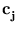,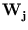,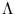j, and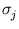. In addition, both methods provide the same error function (3.2) for each unit defined on these variables. The minimum of this error function over all units is an estimate for the squared distance to the distribution of training patterns. This estimate will be the basis for the pattern recall.Next: 4. Abstract recurrent neural Up: 3. Mixture of local Previous: 3.4.2 Results
Heiko Hoffmann
2005-03-22